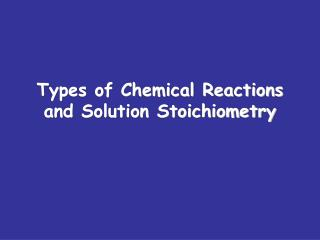DownloadDownload PresentationTypes of Chemical Reactions and Solution Stoichiometry

# Types of Chemical Reactions and Solution Stoichiometry

Télécharger la présentation## Types of Chemical Reactions and Solution Stoichiometry

- - - - - - - - - - - - - - - - - - - - - - - - - - - E N D - - - - - - - - - - - - - - - - - - - - - - - - - - -
##### Presentation Transcript

1. Types of Chemical Reactionsand Solution Stoichiometry

2. Oxidation and Reduction (Redox) • Electrons are transferred • Spontaneous redox rxns can transfer energy • Electrons (electricity) • Heat • Non-spontaneous redox rxns can be made to happen with electricity

3. Oxidation and Reduction An old memory device for oxidation and reduction goes like this… LEO says GER LoseElectrons = Oxidation GainElectrons = Reduction

4. Oxidation Reduction Reactions(Redox) Each sodium atom loses one electron: Each chlorine atom gains one electron:

5. LEO says GER : Lose Electrons = Oxidation Sodium is oxidized Gain Electrons = Reduction Chlorine is reduced

6. Rules for Assigning Oxidation NumbersRules 1 & 2 • The oxidation number of any uncombined element is zero 2. The oxidation number of a monatomic ion equals its charge

7. Rules for Assigning Oxidation NumbersRules 3 & 4 3.The oxidation number of oxygen in compounds is -2 4. The oxidation number of hydrogen in compounds is +1

8. Rules for Assigning Oxidation Number Rule 5 5. The sum of the oxidation numbers in the formula of a compound is 0 2(+1) + (-2) = 0 H O (+2) + 2(-2) + 2(+1) = 0 Ca O H

9. Rules for Assigning Oxidation NumbersRule 6 6. The sum of the oxidation numbers in the formula of a polyatomic ion is equal to its charge X + 4(-2) = -2 S O X + 3(-2) = -1 N O  X = +5  X = +6

10. Reducing Agents and Oxidizing Agents • The substance reduced is the oxidizingagent..loses electron • The substance oxidized is the reducingagent..gains electron Sodium is oxidized – it is the reducing agent Chlorine is reduced – it is the oxidizing agent

11. Trends in Oxidation and Reduction • Active metals: • Lose electrons easily • Are easily oxidized • Are strong reducing agents • Active nonmetals: • Gain electrons easily • Are easily reduced • Are strong oxidizing agents

12. Redox Reaction Prediction #1

13. Redox Reaction Prediction #2

14. Not All Reactions are Redox Reactions Reactions in which there has been no change in oxidation number are not redox rxns. Examples:

15. BALANCING OXIDATION/REDUCTION REACTIONS • Split the equation into two halves (oxidation/reduction). • Balance one of the half–equations with respect to both atoms and charge. • Balance the other half • Balance the half-equations to eliminate electrons

16. VERY IMPORTANT! • If you mix two volumes, to find the new molarity divide by total volume!